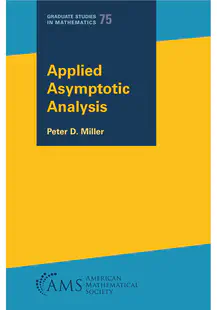# Math 671--Asymptotic Methods

### Course Info

Almost everything is on the course canvas page.

### Why do I have this webpage then?

Because Canvas is not very good at typesetting long stretches of math, like this equation that may have a boundary layer

$$\begin{gathered} \epsilon y’’(x) + y’ + e^{-y} = 0, \, 0< x< 1 \\ y(0)=1, \, y(1) = -1. \end{gathered}$$

and at highlighting code (which isn’t working, but at least it’s in a good font), like this Fibonacci sequence calculation in MATLAB

% Good code has comments
n = 5;
a = zeros(1,n);
for k = 3:n
a(k) = a(k-1) + a(k-2);
end
plot(1:n,a)


and sometimes I like to share math and computer code with my classes.